## Partial summation

### Quick description

Suppose that you are trying to bound the size of a sum of a product of two functions, one reasonably smooth and the other reasonably oscillating, and suppose that you believe that the oscillations give rise to cancellation that causes the sum to be small. Then a discrete analogue of the "use integration by parts to exploit cancellation" trick may well give rise to an efficient way of proving that your belief is correct.

### Prerequisites

Basic real analysis. Complex numbers.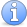Incomplete This article is incomplete. It would be very nice to have more examples, both for evaluating sums and for estimating them.

### Example 1: Abel summation

Letbe a complex number, of modulusbut not equal to, and let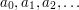be a decreasing sequence of positive real numbers tending to zero. Then the sum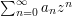converges.

How might we prove this? Why do we even believe it?

One can give a geometrical answer to the second question. If you plot the points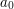,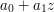,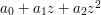,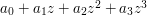, and so on, drawing straight line segments between them, then you obtain a piecewise linear curve that seems to spiral inwards to a point. (The closeris to, the bigger this "spiral" tends to be.)

How about a rigorous proof? Well, the observation on which the proof is based is that the set of numbers of the form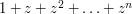is bounded. Indeed, the formula for summing a geometric progression tells us that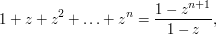which has modulus at most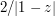.

Since we know how to work out sums of the above form, it makes sense to try to use this information to investigate the sum, which we do by breaking it up into sums of the form we like. We can set aside the convergence issues for now and just look at the sum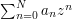. In order to split this up into polynomials with constant coefficients, we begin by noting that the sequence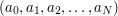can be split up as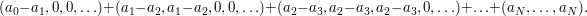The best motivation for this splitting comes from drawing a picture of the "graph" of the sequence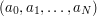, which we are chopping up horizontally.Attention This article is in need of attention. If anyone knows how to produce a nice picture for this it will really help.

This suggests the following way of rewriting the sum;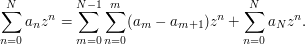To check that this rewriting is correct, we can use the old trick of interchanging the order of summation.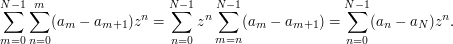Adding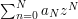to this gives us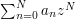as we wanted.

Once we have this decomposition of the sum, the estimate above for sums of geometric progressions involvingtells us that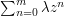has modulus at most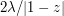for any positive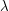. Therefore, we see instantly that the rewritten sum has modulus at most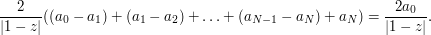This calculation has already illustrated the main idea of partial summation. However, let us finish the proof. The same argument shows that the partial sum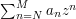has modulus at most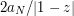. Since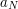is assumed to tend to zero, this proves that the sequence of partial sums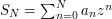is Cauchy, and therefore it converges, which is what we wanted to prove.

### General discussion

Analogy with integration by parts. At the beginning of this article, we claimed that partial summation was the discrete analogue of integration by parts. What is the connection?

To answer this question, let us write down a general principle (together with its proof) that encapsulates what we have done. (The notational conventions will be somewhat different, however.)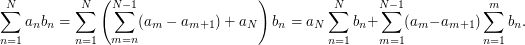Now let us write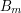for the partial sum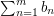. Then this identity becomes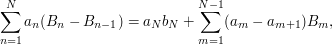which is closely analogous to the formula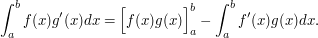We are simply replacing the derivative by the difference operator and integration by summation. (We are also restricting attention to functions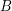such that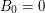, which is why the first terms on the right-hand side seem to be slightly different, but in principle one could generalize the discrete formula.)

When is partial summation useful? One answer to this is rather like the answer in the case of integration by parts. If we have a sum of the form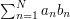that we find hard to evaluate or estimate, we may be able to simplify it by replacing the sequence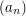by a difference sequence and the sequence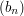by a sequence of partial sums.

If that seems a bit tautologous, then here is a slightly more restricted answer. One circumstance where partial summation is useful is when one can get good upper bounds for the partial sums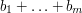and also good upper bounds for the sum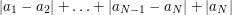. This often occurs if the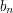oscillate a lot and the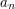vary fairly smoothly.

### Example 2: a simple statement that implies the Riemann hypothesis

A recent paper of Maier and Montgomery refers to a well-known result of Littlewood, that the Riemann hypothesis is equivalent to the statement that the partial sums of the Möbius function do not grow much faster than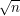. They include the following sentence, having made the assumption that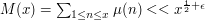. (This means that for every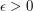there is a constant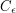such that we have the bound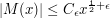.)

"The converse is trivial, since this estimate, by partial summation, implies that the series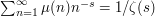converges for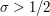."

[H. Maier and H. L. Montgomery, The sum of the Möbius function, Bulletin LMS, Volume 41, 2009, p. 213.]

Since the seriescannot converge if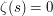, this tells us that the Riemann hypothesis would follow if one could prove the above bound for partial sums of the Möbius function.

Here is the argument that Maier and Montgomery had in mind (which is indeed trivial once one is used to partial summation). We want to prove that the infinite sum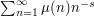converges. This sum is made out of a function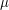that we think behaves rather randomly, and a function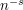that decays in a nice smooth way. The "randomness" ofleads us to believe that its partial sums do not grow too fast (indeed, this is our hypothesis), so it makes obvious sense to use partial summation, "integrating" thepart and "differentiating" thepart. This gives us the identity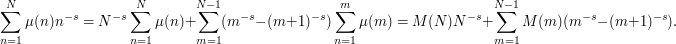Suppose that the real partofis greater than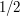and letbe a real number strictly betweenand. Then our hypothesis tells us that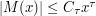for every, where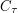is a constant that depends ononly. Also,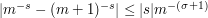, by the mean value theorem. Roughly, the idea here is that the modulus of the derivative of the function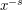never exceeds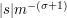on the interval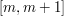. See how to use the mean value theorem for more details about this kind of argument. With these two estimates and the identity above, we find that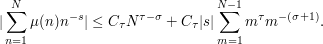The first term tends to zero as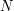tends to infinity, and the second term is bounded because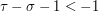. Therefore, the infinite sumconverges.

If you want to know why, then see the article on Möbius inversion.

### General discussion

Note that the infinite sumdoes not converge absolutely, since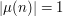far too often. So the convergence exploits cancellation in a crucial way, which is one of the signs that partial summation may be a useful tool.

Note also a difference between Example 1 and Example 2. In Example 1 we managed to replace the original sum of products with a sum of products in which one sequence was bounded and the other absolutely summable, whereas in Example 2 we obtained two new sequences where one tended to infinity, but the other tended to zero fast enough to compensate for that and give rise to a convergent sum.